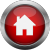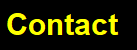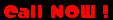The AWG - American Wire Gauge - is used as a standard method of denoting wire diameter, measuring the diameter of the conductor (the bare wire) with the insulation removed. The higher the number - the thinner the wire. This AWG table is for a single, solid, round wire. Small gaps between the strands in a stranded wire make it so it has to be slightly larger to keep the same current-carrying capacity and electrical resistance as a solid wire. Because a thick wire has less electrical resistance it will carry more current with less voltage drop than a thin wire. AWG Diameter (mm) Diameter (in) Circular Mils Square (mm2) Resistance (ohm/1000m) 14 1.63 0.064 4110 2.0 8.54 12 2.05 0.081 6530 3.3 5.4 10 2.59 0.10 10380 5.26 3.4 8 3.25 0.13 16510 8.30 2.2 6 4.115 0.17 26240 13.30 1.5 4 5.189 0.20 41740 21.15 0.8 2 6.543 0.26 66360 33.62 0.5 1 7.348 0.29 83690 42.41 0.4 0 8.252 0.33 105600 53.49 0.31 00 (2/0) 9.266 0.37 133100 67.43 0.25 000 (3/0) 10.40 0.41 168800 85.01 0.2 0000 (4/0) 11.684 0.46 211600 107.22 0.16

What good is all this data? I put it here so you can calculate the proper wire size for your
mobile installations. (yes I only included the wire sizes I thought were important)
The formula for voltage drop is:

VD = 2 x K x I x D/CM.

• K = Direct-Current Constant. K represents the dc resistance. (for a 1,000-circular mils conductor that is 1,000 ft long, at an operating temperature of 75şC).
K is 12.9 ohms for copper and 21.2 ohms for aluminum.
• I = Amperes: The load in amperes at 100%
• D = Distance:  Where we specify distances here, we are referring to the conductor length.
• CM = Circular-Mils: The circular mils of the circuit conductor as listed in NEC Chapter 9, Table 8.

Let’s do an example. An amplifier rated 13.8VDC and 75A is wired
to the Batteries with 10 ft lengths of 4 AWG stranded wire.

 Copper Wire K = 12.9 ohms, copper I = 75A D = 10 ft CM = 41740 VD = 2 x 12.9 x 75 x 10 / 41740 =  .46 vdc dropped (So the voltage at the amplifier will be 13.34 vdc) Aluminum Wire K = 21.2 ohms, Aluminum I = 75A D = 10 ft CM = 41740 VD = 2 x 12.9 x 75 x 10 / 41740 =  .76 vdc dropped (So the voltage at the amplifier will be 13.04 vdc)

Just one more to those 16 pill-ers out there using 15 feet of 1/0 wire....this same formula for
350 amps would leave a voltage drop of 1.32 vdc and would leave 12.48 vdc at the amplifier.
1.32vdc x 350A x 65% = 300 watts lost

Reference : National Electrical Code (NEC)954-771-8161 TECH INFO